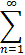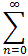#Interactive Real Analysis

Next | Previous | Glossary | Map

## 8.4. Taylor Series

### Example

Which function is represented by the series1/n xn

Our known series with which to start is, once again, the Geometric series. For variety, let's use t as variable:

1/1-t =tn =tn-1

Integrating both sides gives:1/1-t dt =tn-1 dt =tn-1 dt =1/n xn

Thus, the function represented by this series is:1/n xn =1/1-t dt = -ln(1-x)1/n xn f(x) = -ln(1-x)
Next | Previous | Glossary | Map